×

Search anything:

# Deep Belief Network

#### Machine Learning (ML)Get this book -> Problems on Array: For Interviews and Competitive Programming

Backpropagation is a supervised learning algorithm that provides an effective way to learn nonlinear characteristics in the hidden layers of a multi-layer perceptron (MLP). However, as the network size increases, the algorithm fails to optimise network weights leading to poor feature selection and slowing down the learning process. In addition, it requires considerable labeled data on training, which is costly to obtain. Deep Belief Networks are unsupervised learning models that overcome these limitations. We will explore them in details in this article at OpenGenus.

# Table of Content

1. Definition
2. The learning procedure
3. DBN Applications
4. Generating images using a DBN
5. DBN limitations
6. Conclusion

# 1. Definition

A Deep Belief Network (DBN) is a probabilistic generative model that is composed of a stack of latent variables called also hidden units.

• The top two layers have undirected, symmetric connections and form an associative memory.
• The layers below have directed top-down connections between them.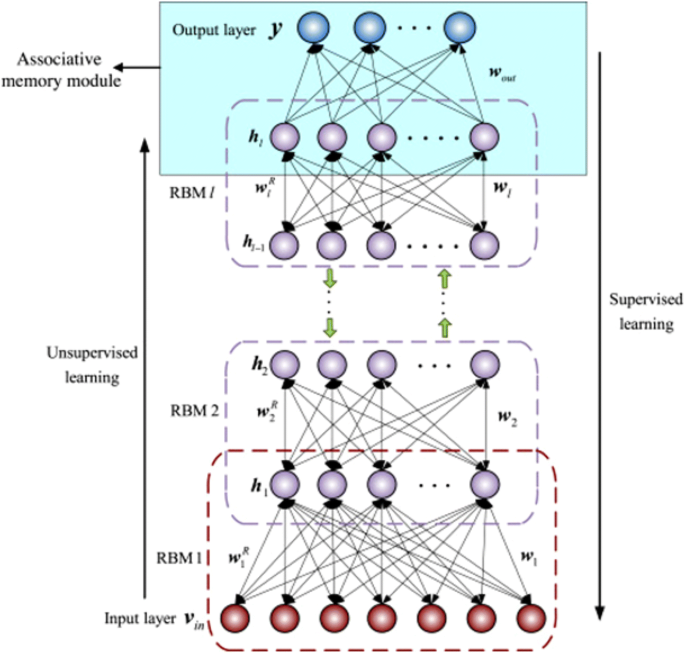Deep Belief Network Architecture 

The building block of a deep belief network is a simple unsupervised networks that can be either a restricted Boltzmann Machine or an Auto-encoder.

DBNs have two important computational properties  :

• First, there is an efficient algorithm to learn the top-down weights that exists between the layers of the network
• Second, after training the weights, it is possible to infer the values of the latent variables by a bottom-up pass starting with an observed data vector in the bottom layer.

# 2. The learning procedure

The learning algorithm of a deep belief network is divided in two steps:

1. Layer-wise Unsupervised Learning.
2. Fine-Turning.

## Layer-wise Unsupervised Learning:

This is the first step of the learning process, it uses unsupervised learning to train all the layers of the network. The algorithm describing this phase is as follow :

1. Train the first layer as an RBM that models the raw input as its visible layer.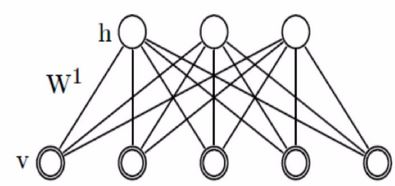1. Once the layer has been trained, fix its weights W and infer the outputs of this layer from the training data.
2. Use the resulted output as inputs for the next layer.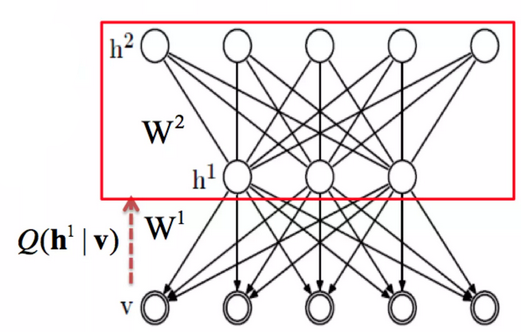1. Keep repeating steps 1,2 and 3 until all the RBM layers has been trained.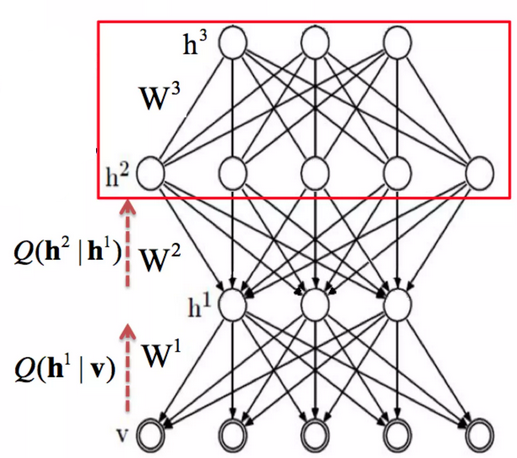## Fine-tuning:

This step is needed to do discriminative tasks. For example if we need the DBN to perform a classification task, we need to add a suitable classifier to its end, such as Backpropagation Network. The weight matrix of the whole network is revised by the gradient descent algorithm, this leads to slightly changing the parameters of the RBMs.
In this way the trained DBN will not be easily damaged.

# 3. DBN Applications

Deep Belief Networks have been used in a wide range of applications :

1. Generating images
2. Image processing like super-resolution images
3. Image recognition
4. Video recognition
5. Motion capture
6. Non-linear dimensionality reduction
7. Natural language processing

# 4. Classifying the MNIST dataset using DBN

Tensorflow provides an implementation to a DBN architecture using an RBM as its building block. We will apply this implementation on the MNIST dataset.
The link to the github repository can be found here.

1. We start by importing the necessary packages
``````from sklearn.datasets import load_digits
from dbn.tensorflow import SupervisedDBNClassification
import numpy as np
import matplotlib.pyplot as plt
from sklearn.model_selection import train_test_split
from sklearn.metrics import accuracy_score
``````
1. Load the MNIST dataset, scale the data and split it into training and test sets.
``````# Loading dataset
X, Y = digits.data, digits.target

# Data scaling
X = (X / 16).astype(np.float32)

# Splitting data
X_train, X_test, Y_train, Y_test = train_test_split(X, Y, test_size=0.2, random_state=0)
``````
1. Create the DBN classifier and launch the training
``````# Create the model
classifier = SupervisedDBNClassification(hidden_layers_structure=[256, 256],
learning_rate_rbm=0.05,
learning_rate=0.1,
n_epochs_rbm=10,
n_iter_backprop=100,
batch_size=32,
activation_function='relu',
dropout_p=0.2)
# Training
classifier.fit(X_train, Y_train)
``````
1. Evaluate your model on the Test set
``````Y_pred = classifier.predict(X_test)
print('Done.\nAccuracy: %f' % accuracy_score(Y_test, Y_pred))
``````

Accuracy: 0.983333

1. Plot some images from the Test set and their predicted labels
``````images = [X_test[i] for i in range(10)]
images = list(map(lambda x: x.reshape(8,8), images))

labels =  [Y_test[i] for i in range(10)]
predictions =  [Y_pred[i] for i in range(10)]

fig, axes = plt.subplots(2,5, figsize=(15,8))

for i in range(10):
row = i//5
col = i % 5
axes[row][col].imshow(images[i])
axes[row][col].set_title(f'label: {labels[i]} ,pred:{predictions[i]}')
``````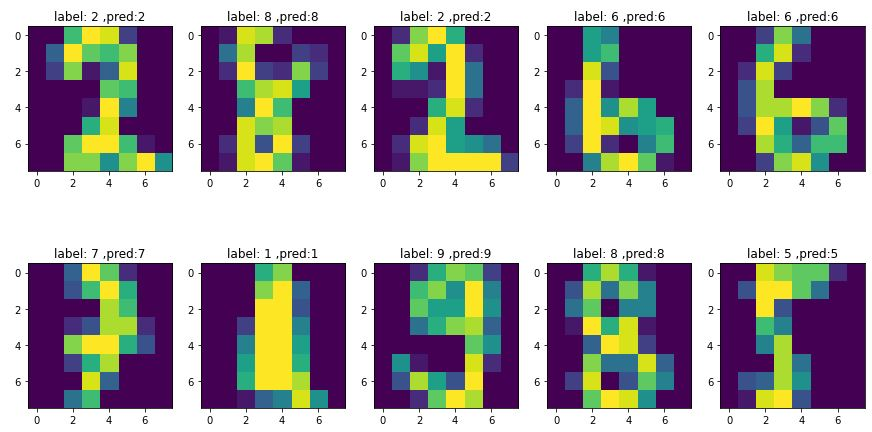You can find the link to the full code here

# 5. DBN advantages and limitations

• They are less computationally expensive because they grow linearly in computation complexity with the number of layers rather than exponentially as with feed forward neural networks.
• They are less vulnerable to the vanishing gradient problem.

## Limitations

Although deep belief networks have great applications, they have also some limitations:

• DBN has expensive hardware requirements.
• DBN requires huge amount of data to perform better.
• DBN has complex data models.

# 6. Conclusion

• Deep Belief Networks are generative models that can be seen as a stack of Restricted Boltzmann Machine (RBM).
• Deep Belief Networks are trained one layer at a time by taking the outputs from one layer, when they are being inferred from training data, as the input for the next layer.
• In order to perform discriminative fine-tuning, a final layer is added on top of the last RBM layer to represent the outputs and the backpropagating error derivatives.
• Deep Belief Networks address the limitations with classical neural networks.
• Deep Belief Networks has many applications in computer vision, signal processing and natural language processing.

 KAUR, Manjit et SINGH, Dilbag. Fusion of medical images using deep belief networks. Cluster Computing, 2020, vol. 23, no 2, p. 1439-1453.
 HINTON, Geoffrey E. Deep belief networks. Scholarpedia, 2009, vol. 4, no 5, p. 5947.

#### CHERIFI Imane

Cherifi Imane holds a B.Sc in Computer Science from Ecole Nationale Supérieure d'Informatique (ESI) and has been an intern at LMCS (Laboratoire des Méthodes de Conception des Systèmes) and OpenGenus.